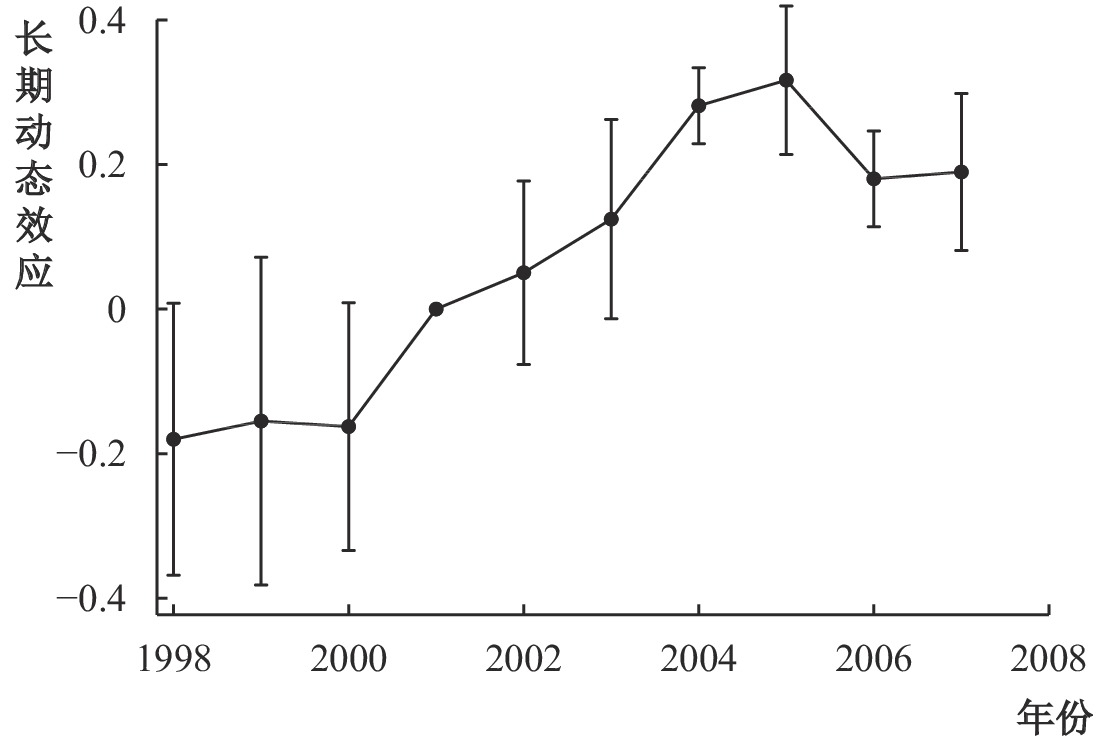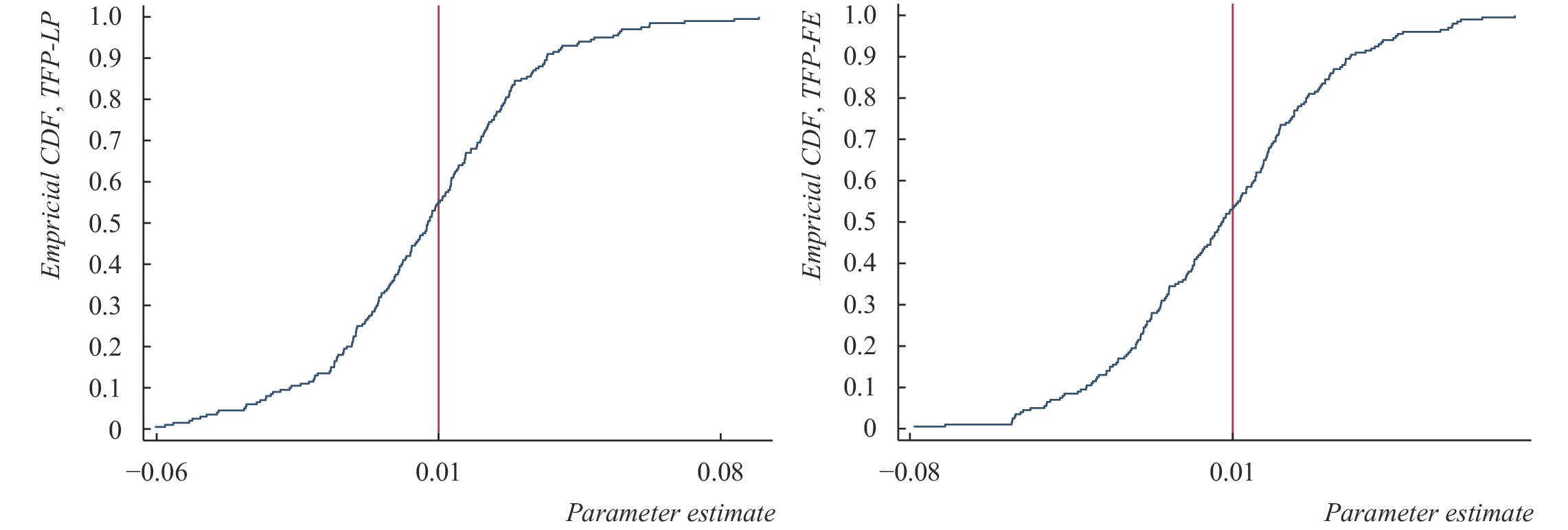﻿ 区域减税政策与企业生产率：学习效应与选择效应《财经研究》
2021第47卷第10期

, 李光勤2,
1. 烟台大学 经济管理学院，山东 烟台 264005;
2. 安徽财经大学 国际经济贸易学院，安徽 蚌埠 233030;
3. 鲁东大学 商学院，山东 烟台 264025

Regional Tax Reduction Policy and Enterprise Productivity：Learning Effect and Selection Effect
, Li Guangqin2,
1. School of Economics and Management，Yantai University，Yantai 264005，China;
2. School of International Trade and Economics，Anhui University of Finance & Economics，Bengbu 233030，China;
Summary: It is of great practical significance for policy-making to accurately identify the effect and mechanism path of regional tax reduction policy on enterprise productivity. China is striving to achieve the “two centenary” goals, which requires the adoption of regional policies to achieve economic development efficiency change and regional coordinated development. In this context, taking the tax reduction policy in the western development region as the research object, this paper explores the implementation effect of regional tax reduction policy on enterprise productivity based on the new economic geography, discusses the mechanism path from learning effect and selection effect, and analyzes the heterogeneity characteristics of enterprises with different productivity. First of all, based on the new economic geography model, this paper increases the parameters of preferential tax policy, theoretically analyzes the mechanism of regional tax reduction policy on enterprise productivity, and puts forward the important inference on the basis of theoretical analysis. Combined with the theoretical inference and the specific implementation background of tax reduction policy in western development, this paper puts forward the research hypothesis that the regional tax reduction policy affects the productivity of enterprises from the two paths of learning effect and selection effect. Secondly, based on the theoretical model and the research hypothesis, this paper takes the regional tax reduction policy of western development as a quasi-natural experiment, and uses the industrial enterprise database data from 1998 to 2007 to build a difference-in-difference (DID) model. Combined with the effective tax rate of the actual income tax, it builds a difference-in-difference-in-difference (DDD) model to verify the impact of the tax reduction policy of western development on the productivity of different tax enterprises. The results show that: On average, the regional tax reduction policy has a significant promoting effect on enterprise productivity. The robustness of the model is tested by the placebo method. Thirdly, we use the kernel density function and quantile estimation to find that the tax reduction policy has heterogeneous effects on enterprises with different productivity, and then apply the heterogeneous enterprise selection model to estimate. The results show that low productivity enterprises are more sensitive to policies and have the advantage of efficiency improvement, which makes the distribution of productivity of enterprises affected by policies more concentrated and the efficiency of resource allocation greatly improved. The conclusion of this paper has a quantitative and concrete effect on the formulation of regional tax reduction policy, and can provide empirical evidence for the accurate formulation of regional tax reduction policy.
Key words: western development    tax reduction policy    enterprise productivity    learning effect    selection effect

（一）理论模型

1. 消费者行为

 $\mathop {{\rm{max}}}\nolimits_{{q_i}\left( v \right),v \in \left[ {{\rm{0}},{\rm{1}}} \right];{Z_i}} {U_i} \equiv \alpha \int_0^1 {{q_i}\left( v \right)} dv - \frac{\beta }{2}\int_0^1 {{{\left[ {{q_i}\left( v \right)} \right]}^2}} dv - \frac{\gamma }{2}{\left[ {\int_0^1 {{q_i}\left( v \right)} dv} \right]^2} + {Z_i}$ (1)
 $\mathrm{s}.\mathrm{t}.{\int }_{0}^{1}{{p}_{i}\left(v\right)q}_{i}\left(v\right)dv+{p}_{i}^{z}{Z}_{i}={y}_{i}+{p}_{i}^{z}\overline {{Z_0}}$ (2)

2. 生产者行为

 ${C}_{i}^{le}\left(q\right)=mq,{C}_{i}^{he}\left(q\right)=0$ (3)

3. 减税政策对不同生产率企业的影响

 ${\pi }_{2}^{he}=(1-\mathrm{\lambda })({p}_{22}^{he}+t){q}_{22}^{he}+\mathrm{\lambda }({p}_{21}^{he}-\tau +t){q}_{21}^{he}$ (4)
 ${\pi }_{2}^{le}=(1-\mathrm{\lambda })({p}_{22}^{le}+t-m){q}_{22}^{le}+\mathrm{\lambda }({p}_{21}^{le}-\mathrm{\tau }+t-m){q}_{21}^{le}$ (5)

 $\Delta {\pi }^{le}\left({s}_{le},{s}_{he},\lambda \right)={\pi }_{1}^{le}-{\pi }_{2}^{le}=\frac{1}{2}\varnothing \left[a-\left(b+c\right)\left(m+\frac{\tau }{2}-\frac{t}{2}\right)\right]$ (6)
 $\Delta {\pi }^{he}\left({s}_{le},{s}_{he},\lambda \right)={\pi }_{1}^{he}-{\pi }_{2}^{he}=\frac{1}{2}\varnothing \left[a-\left(b+c\right)\left(\frac{\tau }{2}-\frac{t}{2}\right)\right]$ (7)

 $\varnothing =\left[\lambda \left(\tau -t\right)-\left(1-\lambda \right)\left(t+\tau \right)\right]+c\omega$
 \begin{aligned} \omega = & \lambda \left(\tau -t\right){P}_{1}-\left(1-\lambda \right)\left(t+\tau \right){P}_{2}\\ = & \frac{\left[\lambda \left(\tau -t\right)-\left(1-\lambda \right)\left(t+\tau \right)\right]\left[a+m\left(b+c\right)\left(1-\mu \right)\right]+\left[\lambda {\left(\tau -t\right)}^{2}+\left(1-\lambda \right)t\left(t+\tau \right)\right]\left(b+c\right)}{2b+c}\\ & -\frac{\left(b+c\right)\left[\lambda {\left(\tau -t\right)}^{2}+\left(1-\lambda \right){\left(t+\tau \right)}^{2}\right]+\left[\mu {s}_{he}+(1-\mu ){s}_{le}\right]}{2b+c} \end{aligned}

（二）研究假说

（一）识别策略设计

（二）模型设定与变量说明

 \begin{aligned} {TFP}_{ickt}=& \alpha {Treat}_{i}\times {ETR}_{ickt}\times YR2001+{\alpha }_{1}{Treat}_{i}\times {ETR}_{ickt}+{\alpha }_{2}{ETR}_{ickt}\times YR2001\\ & +{\alpha }_{3}{Treat}_{i} \times YR2001+{{\alpha }_{4}Treat}_{i}+{\alpha }_{5}{ETR}_{ickt}+{\alpha }_{6}YR2001 +\beta X+{\mu }_{i}+{\lambda }_{ck}+{\xi }_{kt}+{\varepsilon }_{ickt} \end{aligned} (8)

${TFP}_{ickt}$ 为被解释变量，表示t年城市c行业k中第i个企业的生产率，借鉴鲁晓东和连玉君（2012）的研究，本文使用LP方法计算得到TFP_LP。此外，本文还使用索罗残差固定效应方法重新计算了企业生产率TFP_FE进行稳健性检验。 ${Treat}_{i}\times {ETR}_{ickt}\times YR2001$ 为本文的核心解释变量，其中 ${ETR}_{ickt}$ 表示t年城市c行业k中第i个企业的所得税实际有效税率。Treat为西部大开发减税政策的哑变量，如果企业在政策边界线以西则赋值为1，否则赋值为0。由于减税政策的开始时间为2001年，本文将整个样本按照2001年前后进行划分，YR2001为1表示政策实施后，取值为0表示政策实施之前。

X表示一系列控制变量，根据企业生产率影响因素的现有文献，本文在实证模型中控制了以下因素：企业规模（ln_size）、资产负债率（lev）、资产报酬率（roa）、出口规模（ln_ex）以及获得的政府补贴收入（ln_faid）。

 变量名称 变量符号 变量定义 均值 标准差 被解释变量 企业全要素生产率1 TFP_LP 使用LP方法计算的工业企业全要素生产率 6.490 1.401 企业全要素生产率2 TFP_FE 使用FE方法计算的工业企业全要素生产率 0.832 1.147 政策变量 西部大开发政策 Treat 企业是否受到西部大开发政策影响，是为1 0.286 0.021 政策实施时间 YR2001 样本时间在2001年之后为1，之前为0 0.686 0.128 企业实际税负 ETR 企业支付的企业所得税除以营业收入（%） 0.114 0.166 控制变量 企业规模 ln_size 企业期末总资产取对数 9.742 1.532 资产负债率 lev 企业期末负债除以资产 0.551 0.266 资产报酬率 roa 当期利润总额除以总资产 0.098 0.450 出口规模 ln_ex 企业出口额加1后取对数 1.278 3.263 政府补贴 ln_faid 企业获得的政府补贴收入加1后取对数 0.602 1.892

（三）数据说明与描述性统计

 变量 时间 有政策边界市均值 无政策边界市均值 单重差分均值（标准差） 双重差分均值（标准差） TFP_LP 2001年之前 5.610 5.938 −0.327***（0.021） 0.206***（0.025） 2001年之后 6.600 6.721 −0.122***（0.012） TFP_FE 2001年之前 0.235 0.364 −0.129*** （0.016） 0.083***（0.019） 2001年之后 0.957 1.003 −0.047*** （0.009）

（一）基准回归分析

 （1） （2） （3） （4） （5） （6） （7） TFP_LP TFP_LP TFP_LP TFP_FE 平衡面板 税收优惠行业 非税收优惠行业 Treat×YR2001×ETR 0.2102*** 0.2331*** 0.1750*** 0.1497*** 0.2106** 0.1827*** 0.0630 （0.0640） （0.0705） （0.0486） （0.0516） （0.1002） （0.0661） （0.0544） Treat×YR2001 0.113*** 0.0733*** 0.108** 0.0642*** 0.184*** 0.231*** −0.265*** （0.0310） （0.0226） （0.0439） （0.0223） （0.0449） （0.0570） （0.0665） Treat×ETR −0.0170 −0.00507 −0.00707* −0.00539 −0.0317*** 0.701*** 0.0518 （0.0123） （0.00713） （0.0040） （0.00713） （0.0123） （0.173） （0.0880） YR2001×ETR −0.0171 −0.00474 0.0365 −0.00549 −0.0280** −0.000908 0.0566 （0.0120） （0.00677） （0.0237） （0.00672） （0.0123） （0.0126） （0.0578） ETR 0.0170 0.00498 0.00631* 0.00563 0.0300** 0.0118 −0.00228* （0.0119） （0.0068） （0.0037） （0.0067） （0.0122） （0.0120） （0.0014） ln_size 0.383*** 0.415*** 0.267*** 0.374*** 0.425*** 0.280*** （0.0060） （0.0211） （0.0057） （0.0095） （0.0143） （0.0185） lev −0.0721*** −0.105 0.0201 −0.0136 −0.176*** −0.0729 （0.0194） （0.0718） （0.0193） （0.0303） （0.0446） （0.0539） roa 1.5260*** 2.6710*** 1.442*** 1.3310*** 1.4300*** 1.6590*** （0.0852） （0.1820） （0.0826） （0.1100） （0.1720） （0.0893） ln_ex 0.0136*** 0.0055 −0.0045*** 0.0100*** 0.0243*** 0.0226*** （0.0016） （0.0046） （0.0017） （0.00328） （0.00387） （0.0064） ln_fadi −0.0042* −0.0084 −0.0087*** −0.00337 −0.0115** 0.0018 （0.0023） （0.0058） （0.0022） （0.0037） （0.0051） （0.0073） Constant 6.533*** 6.515*** 1.815*** 1.414*** 1.227*** 1.830*** 1.247*** （0.0084） （0.0096） （0.0453） （0.0440） （0.1720） （0.0448） （0.1710） 样本数 88 038 83 421 79 096 79 096 8 235 43 866 35 204 R2 0.866 0.860 0.835 0.859 0.789 0.841 0.856 Firm FE 未控制 控制 控制 控制 控制 控制 控制 City×Industry FE 未控制 控制 控制 控制 控制 控制 控制 Industry×Year FE 未控制 控制 控制 控制 控制 控制 控制 注：模型中还控制了Treat和YR2001两个变量，由于与时间固定效应和企业固定效应存在共线性，表中没有汇报这两个变量的估计系数。括号内为在城市层面聚类并修正异方差后的稳健标准误，***、**和*分别表示1%、5%和10%的显著性水平。图 1 西部大开发减税政策的长期动态效应

（二）安慰剂检验

 ${TFP}_{ickt}=\alpha {Random}_{it}+{\beta }_{n}X+{\mu }_{i}+{\lambda }_{ck}+{\xi }_{kt}+{\varepsilon }_{ickt}$ (9)

 Panel A：安慰剂检验 （1） （2） TFP_LP TFP_FE 安慰剂效应 0.0300 −0.0200 （0.0185） （0.0420） 控制变量 控制 控制 Firm FE 控制 控制 City×Industry FE 控制 控制 Industry×Year FE 控制 控制 Panel B：200次安慰剂检验的结果分布 被解释变量 显著 未通过5%水平的 显著性检验 大于0 小于0 TFP_LP 19 9 172（86%） TFP_FE 15 10 175（87.5%）图 2 安慰剂检验回归系数的累积概率分布图

（一）两种效应的初步验证

 90%分位数 75%分位数 中位数 25%分位数 10%分位数 （1） （2） （3） （4） （5） Treat×YR2001×ETR 0.0366* 0.0665*** 0.1163** 0.1482*** 0.2710*** （0.0203） （0.0117） （0.0553） （0.0127） （0.0163） Constant 0.518*** 1.026*** 1.501*** 2.003*** 2.710*** （0.0516） （0.0403） （0.0328） （0.0318） （0.0394） 样本数 79244 79244 79244 79244 79244 控制变量 控制 控制 控制 控制 控制 Firm FE 控制 控制 控制 控制 控制 City×Industry FE 控制 控制 控制 控制 控制 Industry×year FE 控制 控制 控制 控制 控制 注：括号内为在城市层面聚类并修正异方差后的稳健标准误，***、**和*分别表示1%、5%和10%的显著性水平。

（二）异质性企业选择模型

 ${\mathrm{\varnothing } _a}\left( x \right) = {\rm{ln}}\left( {\frac{{\sum\nolimits_b {{Q_{ab}}} \left( x \right)}}{{{l_a}\left( x \right)}}} \right) = {I_a} - {\rm{ln}}\left( x \right)$ (10)

 ${F}_{a}\left(\varnothing \right)=\max\left\{0,\dfrac{\tilde F\left(\frac{\varnothing -{I}_{a}}{{J}_{a}}\right)-{W}_{a}}{1-{W}_{a}}\right\}$ (11)

 ${m}_{\theta }\left(u\right)={\lambda }_{a}\left({r}_{w}\left(u\right)\right)-J{\lambda }_{b}\left(W+\left(1-W\right){r}_{w}\left(u\right)\right)-I, u \in \left[\mathrm{0,1}\right]$ (12)
 $\widetilde {{m_\theta }}\left(u\right)={\lambda }_{b}\left({\tilde r}_{w}\left(u\right)\right)-\frac{1}{J}{\lambda }_{a}\left(\frac{{\tilde r}_{w}\left(u\right)-W}{1-W}\right)+\frac{I}{J}, u \in \left[\mathrm{0,1}\right]$ (13)

 ${r}_{w}\left(u\right)=\max\left\{0,-W/(1-W)\right\} + \left(1-\max\left\{0,-W/\left(1-W\right)\right\}\right)u$
${\tilde r}_{w}\left(u\right)=\max\left\{0,W\right\}+(1-\max\left\{0,W\right\})u$ ${\lambda }_{k}\left(u\right)\equiv {F}_{k}^{-1}\left(u\right)$ ${F}_{k}$ 在概率为u处的生产率分位数点， $k=a,b$ 。采用线性插值法求得概率u对应的生产率分位数点后，便可以得到最终的估计目标函数：

 $\widehat{\theta }=\mathrm{arg}\underset{\theta }{\mathrm{min}}M\left(\theta \right)$ (14)
 $\mathrm{s. t.} \;\;\; M \left(\mathrm{\theta }\right)={\int }_{0}^{1}{\left[{\widehat{M}}_{\theta }\left(u\right)\right]}^{2}du+{\int }_{0}^{1}{\left[{\hat \tilde M}_{\theta }\left(u\right)\right]}^{2}du$ (15)

 ${\int }_{0}^{1}{\left[{\widehat{M}}_{\theta }\left(u\right)\right]}^{2}du\approx \dfrac{1}{2}\sum _{k=1}^{k}\left\{{\left[{\widehat{M}}_{\theta }\left({u}_{k}\right)\right]}^{2}+{\left[{\widehat{M}}_{\theta }\left({u}_{k-1}\right)\right]}^{2}\right\}({u}_{k}-{u}_{k-1})$
 ${\int }_{0}^{1}{\left[{\hat \tilde M}_{\theta }\left(u\right)\right]}^{2}du \approx \dfrac{1}{2}\sum _{k=1}^{k}\Bigr\{{\left[{\hat \tilde M}_{\theta }\left({u}_{k}\right)\right]}^{2}+ {\left[{\hat \tilde M}_{\theta }\left({u}_{k-1}\right)\right]}^{2}\Bigr\}({u}_{k}-{u}_{k-1})$

（三）估计结果分析

 年份 学习效应（I） 异质性效应（J） 选择效应（W） R2 样本数 1998 −0.3614（0.4319） 1.1062***（0.2284） 0.0069（0.3783） 0.9817 6 453 1999 −0.4593（0.4127） 1.1511***（0.2329） 0.0314（0.4235） 0.9725 6 774 2000 −0.4264（0.2953） 1.1755***（0.1970） 0.0249（0.1759） 0.9816 6 636 2001 −0.4139（0.3432） 1.1880***（0.2121） −0.0252（0.3960） 0.9771 6 592 2002 −0.3602（0.3202） 1.1615***（0.1535） −0.0205（0.5570） 0.9879 6 858 2003 0.3065（0.3529） 1.2119***（0.2026） 0.0097（0.3683） 0.9854 7 566 2004 0.0877（0.1796） 1.0172***（0.1240） −0.0301（0.0899） 0.8783 8 976 2005 0.0588***（0.0220） 1.0505***（0.0149） −0.0326***（0.0030） 0.8101 10 250 2006 0.0161*（0.0095） 1.1011***（0.0085） −0.0110***（0.0015） 0.8897 12 561 2007 0.1107***（0.0255） 1.0496***（0.0942） 0.0014（0.0169） 0.9810 13 630 注：括号内为标准差，***、**和*分别表示在1%、5%和10%的水平上显著。

① 1989年我国发布第一份产业政策文件《国务院关于当前产业政策要点的决定》，其中列示了国民经济各领域限制和鼓励的行业。此后，针对我国经济发展过程中出现的各种问题，中央相应出台各种类型政策进行调整和补充。

② 本文关心的减税政策是2001年国务院颁布实施的政策文件《财政部国家税务总局海关总署关于西部大开发税收优惠政策问题的通知》，这是西部大开发区域减税政策的核心政策文件，主要内容包括减税政策适用的地区范围、具体方式及相关《产业目录》等。

③ 如无特殊说明，下文均采用LP方法测算的企业生产率进行回归。

④ 限于篇幅，文中没有展示核密度图，如有需要可向作者索取。

  陈良文, 杨开忠, 沈体雁, 等. 经济集聚密度与劳动生产率差异——基于北京市微观数据的实证研究[J]. 经济学（季刊）, 2008(1): 99–114.  陈钊, 熊瑞祥. 比较优势与产业政策效果——来自出口加工区准实验的证据[J]. 管理世界, 2015(8): 67–80.  范剑勇. 产业集聚与地区间劳动生产率差异[J]. 经济研究, 2006(11): 72–81.  梁琦. 分工、集聚与增长[M]. 北京: 商务印书馆, 2009.  梁琦, 黄卓. 空间经济学在中国[J]. 经济学（季刊）, 2012(3): 1027–1036.  梁琦, 李晓萍, 简泽. 异质性企业的空间选择与地区生产率差距研究[J]. 统计研究, 2013(6): 51–57. DOI:10.3969/j.issn.1002-4565.2013.06.007  梁琦, 李晓萍, 吕大国. 市场一体化、企业异质性与地区补贴——一个解释中国地区差距的新视角[J]. 中国工业经济, 2012(2): 16–25.  刘瑞明, 赵仁杰. 西部大开发: 增长驱动还是政策陷阱——基于PSM-DID方法的研究[J]. 中国工业经济, 2015(6): 32–43.  刘生龙, 王亚华, 胡鞍钢. 西部大开发成效与中国区域经济收敛[J]. 经济研究, 2009(9): 94–105.  刘修岩, 张学良. 集聚经济与企业区位选择——基于中国地级区域企业数据的实证研究[J]. 财经研究, 2010(11): 83–92.  鲁晓东, 连玉君. 中国工业企业全要素生产率估计: 1999-2007[J]. 经济学（季刊）, 2012(2): 541–558.  邵帅, 齐中英. 西部地区的能源开发与经济增长——基于“资源诅咒”假说的实证分析[J]. 经济研究, 2008(4): 147–160.  王永进, 张国峰. 开发区生产率优势的来源: 集聚效应还是选择效应?[J]. 经济研究, 2016(7): 58–71.  赵勇, 魏后凯. 政府干预、城市群空间功能分工与地区差距——兼论中国区域政策的有效性[J]. 管理世界, 2015(8): 14–29.  Almond D, Chen Y Y, Greenstone M, et al. Winter heating or clean air? Unintended impacts of China’s Huai River policy[J]. American Economic Review, 2009, 99(2): 184–190. DOI:10.1257/aer.99.2.184  Arimoto Y, Nakajima K, Okazaki T. Sources of productivity improvement in industrial clusters: The case of the prewar Japanese silk-reeling industry[J]. Regional Science and Urban Economics, 2014, 46: 27–41. DOI:10.1016/j.regsciurbeco.2014.02.004  Baldwin R, Okubo T. Agglomeration, offshoring and heterogenous firms[R]. CEPR Discussion Papers, 2006.  Brandt L, Van Biesebroeck J, Zhang Y F. Creative accounting or creative destruction? Firm-level productivity growth in Chinese manufacturing[J]. Journal of Development Economics, 2012, 97(2): 339–351. DOI:10.1016/j.jdeveco.2011.02.002  Brülhart M, Mathys N A. Sectoral agglomeration economies in a panel of European regions[J]. Regional Science and Urban Economics, 2008, 38(4): 348–362. DOI:10.1016/j.regsciurbeco.2008.03.003  Chetty R, Looney A, Kroft K. Salience and taxation: Theory and evidence[J]. American Economic Review, 2009, 99(4): 1145–1177. DOI:10.1257/aer.99.4.1145  Combes P P, Duranton G, Gobillon L, et al. The productivity advantages of large cities: Distinguishing agglomeration from firm selection[J]. Econometrica, 2012, 80(6): 2543–2594. DOI:10.3982/ECTA8442  Duranton G, Puga D. Micro-foundations of urban agglomeration economies[A]. Henderson J V, Thisse J F. Handbook of regional and urban economics[M]. Amsterdam: Elsevier, 2004.  Forslid R, Okubo T. Spatial sorting with heterogeneous firms and heterogeneous sectors[J]. Regional Science and Urban Economics, 2014, 46: 42–56. DOI:10.1016/j.regsciurbeco.2014.02.005  Gatti R, Love I. Does access to credit improve productivity? Evidence from Bulgaria[J]. Economics of Transition and Institutional Change, 2008, 16(3): 445–465.  Kline P, Moretti E. Local economic development, agglomeration economies, and the big push: 100 Years of evidence from the Tennessee valley authority[J]. The Quarterly Journal of Economics, 2014, 129(1): 275–331. DOI:10.1093/qje/qjt034  Martin L A, Nataraj S, Harrison A E. In with the big, out with the small: Removing small-scale reservations in India[J]. American Economic Review, 2017, 107(2): 354–386. DOI:10.1257/aer.20141335  Nakamura R. Agglomeration economies in urban manufacturing industries: A case of Japanese cities[J]. Journal of Urban Economics, 1985, 17(1): 108–124. DOI:10.1016/0094-1190(85)90040-3  Neumark D, Simpson H. Place-based policies[R]. Working Papers 1410, 2014.  Rice J. Ecological unequal exchange: Consumption, equity, and unsustainable structural relationships within the global economy[J]. International Journal of Comparative Sociology, 2007, 48(1): 43–72. DOI:10.1177/0020715207072159  Saito H. Three essays on firm heterogeneity and regional development[D]. Corvallis: Oregon State University, 2008.  Sveikauskas L. The productivity of cities[J]. The Quarterly Journal of Economics, 1975, 89(3): 393–413. DOI:10.2307/1885259